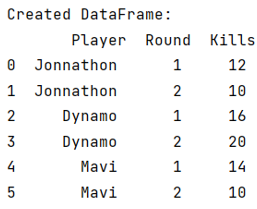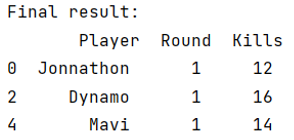# How to get first row of each group in Pandas DataFrame?

Given a Pandas DataFrame, we have to get the first row of each group.
Submitted by Pranit Sharma, on June 04, 2022

Rows in pandas are the different cell (column) values which are aligned horizontally and also provides uniformity. Each row can have same or different value. Rows are generally marked with the index number but in pandas we can also assign index name according to the needs.

## Get first row of each group in Pandas DataFrame

First row means that index 0, hence to get the first row of each row, we need to access the 0th index of each group, the groups in pandas can be created with the help of pandas.DataFrame.groupby() method.

Once the group is created, the first row of the group will be accessed with the nth() method inside which we will pass the index the row which we want, here we want the index 0 of each group.

To work with pandas, we need to import pandas package first, below is the syntax:

```import pandas as pd
```

## Python program to get first row of each group in Pandas DataFrame

Let us understand with the help of an example,

```# Importing pandas package
import pandas as pd

# Create dictionary
d = {
'Player':['Jonnathon','Jonnathon','Dynamo','Dynamo','Mavi','Mavi'],
'Round':[1,2,1,2,1,2],
'Kills':[12,10,16,20,14,10]
}

# Create DataFrame
df = pd.DataFrame(d)

# Display DataFrame
print("Created DataFrame:\n",df)

# Groupby function
result = df.groupby('Player', as_index=False)

# Selecting 1st row of group by result
final = result.nth(0)

# Display final result
print("Final result:\n",final)
```

## Result before grouping## First row of each group along with its index